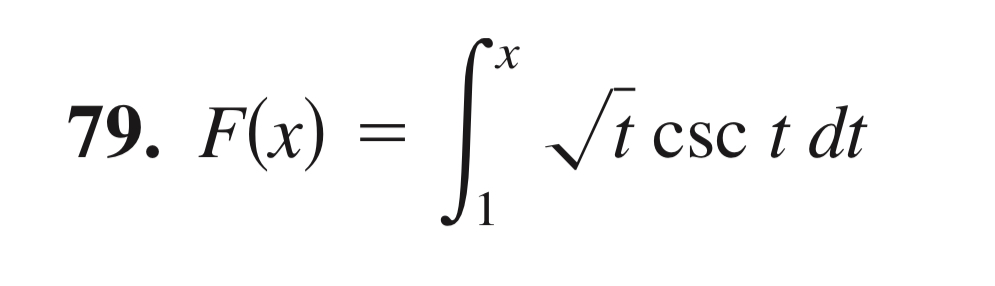79. F(x) =/t CSC t alt

Question

Use the Second Fundamental Theorem of Calculus to find F'(x)help_outlineImage Transcriptionclose79. F(x) = /t CSC t alt fullscreen
Step 1

The mathematical nature of the second fundamental rule of calculus is shown on the white board.

Step 2

In our case, f(t) = t1/2cosec(t)

Hence, F'(x)...

Want to see the full answer?

See Solution

Want to see this answer and more?

Our solutions are written by experts, many with advanced degrees, and available 24/7

See Solution
Tagged in

Calculus